##### How to Convert a String to a Number in PostgreSQL

In PostgreSQL, the “::” operator and CAST operator are used for converting/casting one type to another. Using the TO_NUMBER(), CAST(), and “::” operator, we can convert a string into a numeric type such as an integer, double, or decimal.

The objective of this write-up is to learn how to convert a string into a number using different examples. So, let’s get started!

What is the Need for Converting a String into a Number in PostgreSQL?

Suppose we have a numeric value residing in the string data type (i.e., numeric string), and the need of the moment is to serve number-specific processing. To do so, firstly, we will convert the given strings into numbers. For example, if ‘250’ and '190’ are stored as strings and we have to perform some arithmetic operations on them.

To do so, we will first convert these strings into numeric data types, and then we can perform any operation on these numbers.

How to Convert/Cast a String Into a Number Using CAST Operator?

Follow the below syntax to convert a string into a decimal using the CAST operator:

`SELECT CAST ('expression' AS DECIMAL);`

In the above syntax, the expression represents any numeric string. With the aid of AS clause, the CAST operator will convert the given string to a numeric type.

Example: How to Use CAST Operator in Postgres?

Let’s run the below statement to convert the string to a number using the CAST operator:

`SELECT CAST ('5000.005' AS DECIMAL);`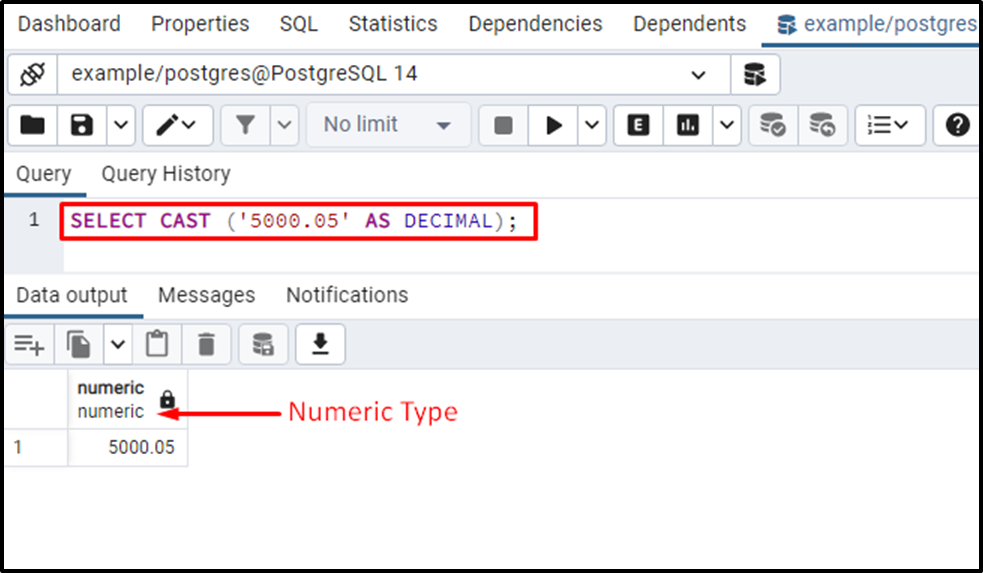The output shows that the given string has been successfully converted into the numeric data type using the CAST operator.

How to Use “::” Operator in PostgreSQL?

Use the below syntax for the ‘string to number’ conversion using the “::” operator:

`SELECT 'expression' :: DECIMAL;`

The above syntax will convert the given string to the decimal/numeric type.

Example: Practical Implementation of the “::” Operator

Consider we have to convert a string “5000.005” to a number type. To do so, use the “::” operator as follows:

`SELECT '5000.05' :: DECIMAL;`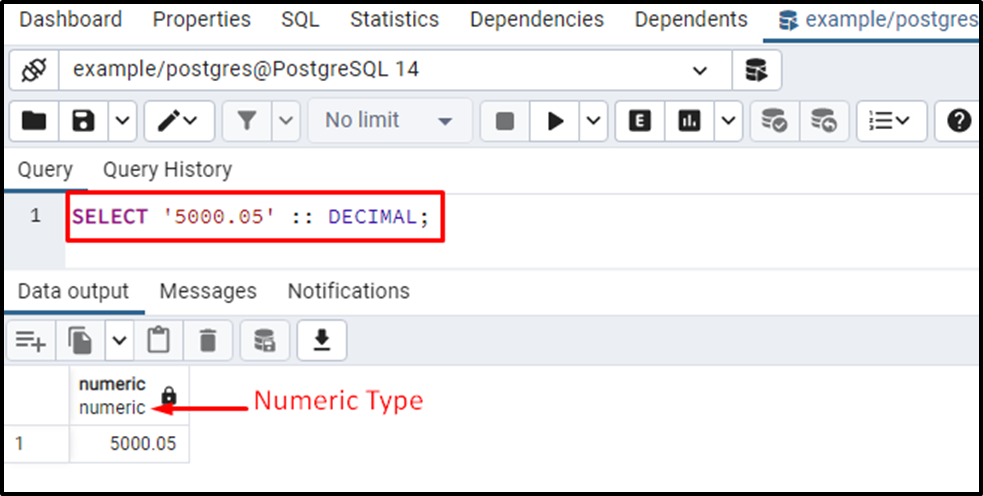The output verifies that the given string “5000.05” has been successfully converted into a numeric type using the “::” operator.

How to Convert a String/Text to an Integer Using CAST Operator?

Here is the syntax for converting a string into an integer using the CAST operator in PostgreSQL:

`SELECT CAST ('expression' AS INTEGER);`

In this syntax, the expression represents any numeric string. With the collaboration of the AS clause, the CAST operator will convert the given string into the integer type.

The CAST operator will generate an error if we try to convert an invalid expression/string into an integer. For example, converting ‘100XYZ’ to integer type will cause an error (because XYZ can’t be converted into a number).

Example #1: How to Use CAST Operator to Convert a String into an Integer/Number?

Execute the below statement to cast a string value “5000” into an int data type:

`SELECT CAST ('5000' AS INTEGER);`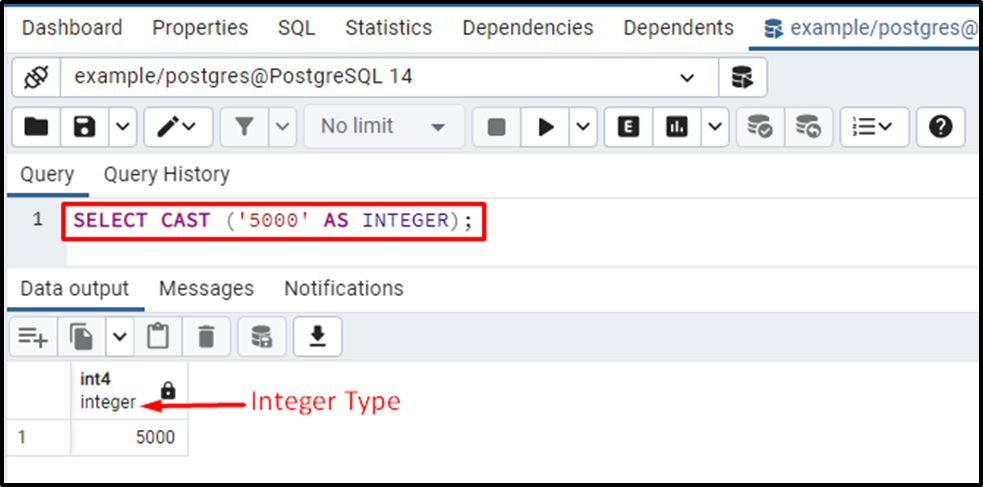The output shows that the given string has been successfully converted into an integer data type.

Example #2: How to Use CAST Operator on Table’s Data?

Suppose we have a table named emp_data. Let’s fetch the table’s data using the SELECT query:

`SELECT * FROM emp_data;`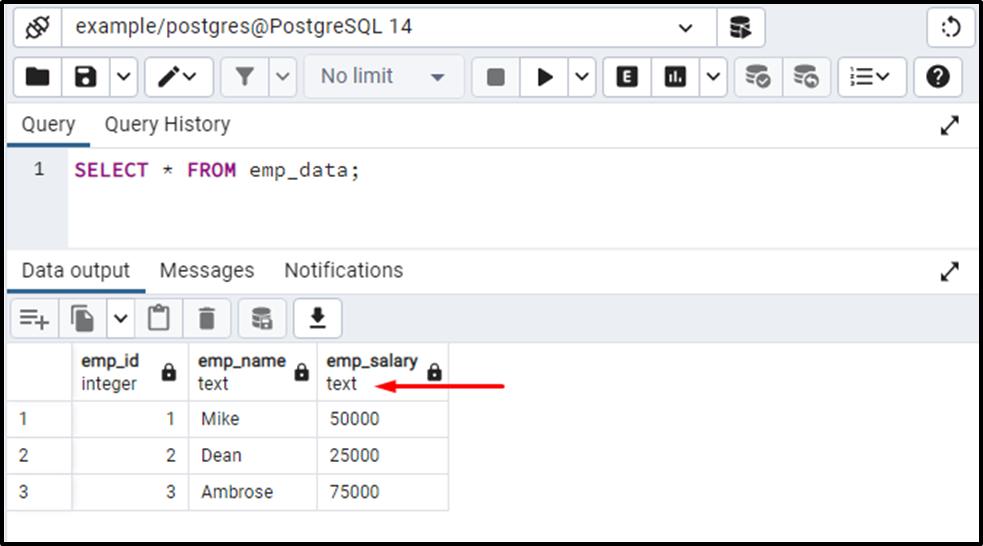You can observe that the data type of emp_salary column is text. Let’s say we need to perform the addition on the “emp_salary” column. To do that, we will use the Postgres built-in function SUM():

```SELECT SUM(emp_salary)
AS total
FROM emp_data;```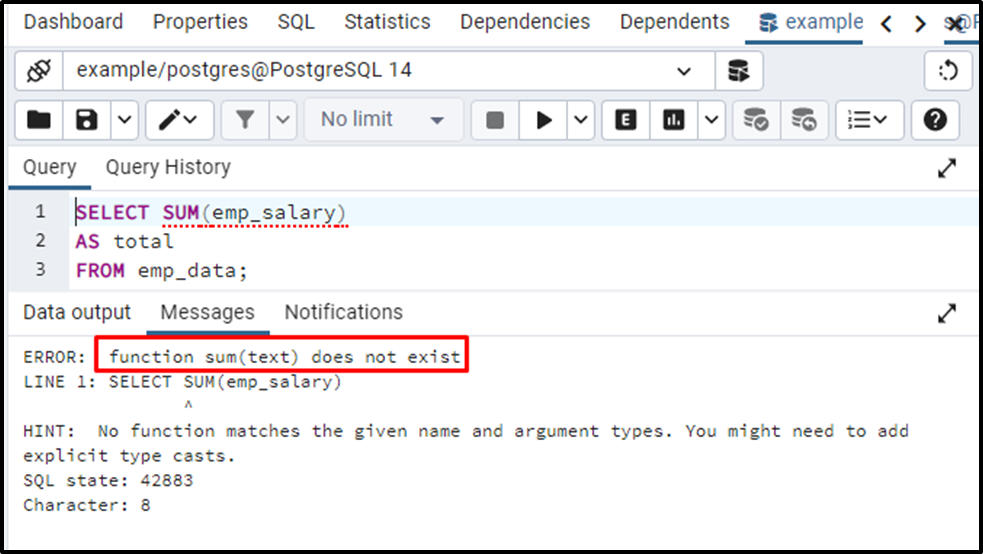When we executed the above query, we encountered an error. Because we can’t perform the addition on the string data.

Suppose we have to perform addition on the emp_salary column. To do that, firstly, we will convert the emp_salary column to the integer data type using the CAST operator:

```SELECT SUM(CAST(emp_salary AS INTEGER))
AS total
FROM emp_data;```

Here, firstly, we utilized the CAST operator to convert the emp_salary column to the desired data type. Next, we utilized a built-in SUM() function to calculate the sum of all the data present in the emp_salary column: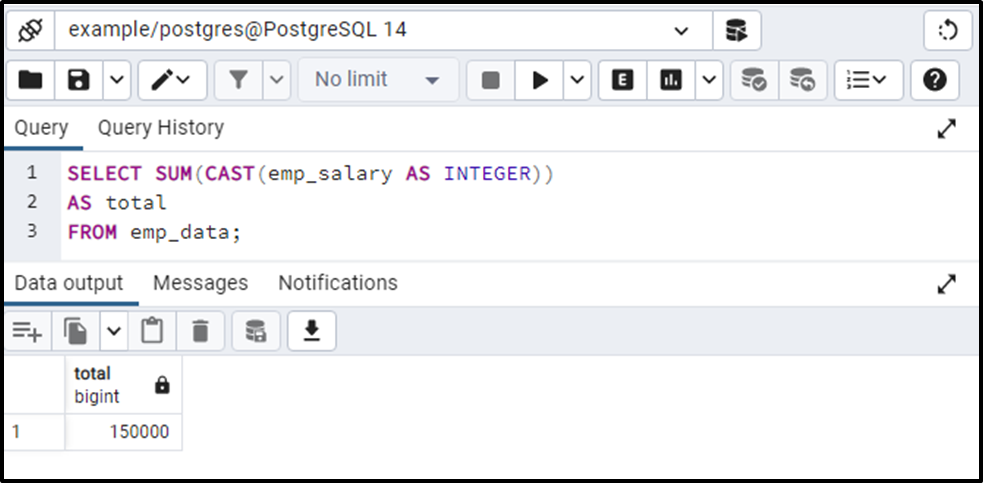This is how you can use the CAST operator on the table’s data to perform different operations.

How to Convert/Cast a String to an INT Using ‘::’ Operator in PostgreSQL?

The “::” operator works the same as the cast operator. Let’s follow the below syntax for converting a string/text to int data type in Postgres:

`SELECT 'Expression':: INTEGER;`

Here, the expression represents any numeric string. The ‘::’ operator will convert the given string into the integer type.

Example #1: How Does the ‘::’ Operator Work in Postgres?

Let’s convert “5000” to an integer type using the “::” operator:

`SELECT '5000'::INTEGER;`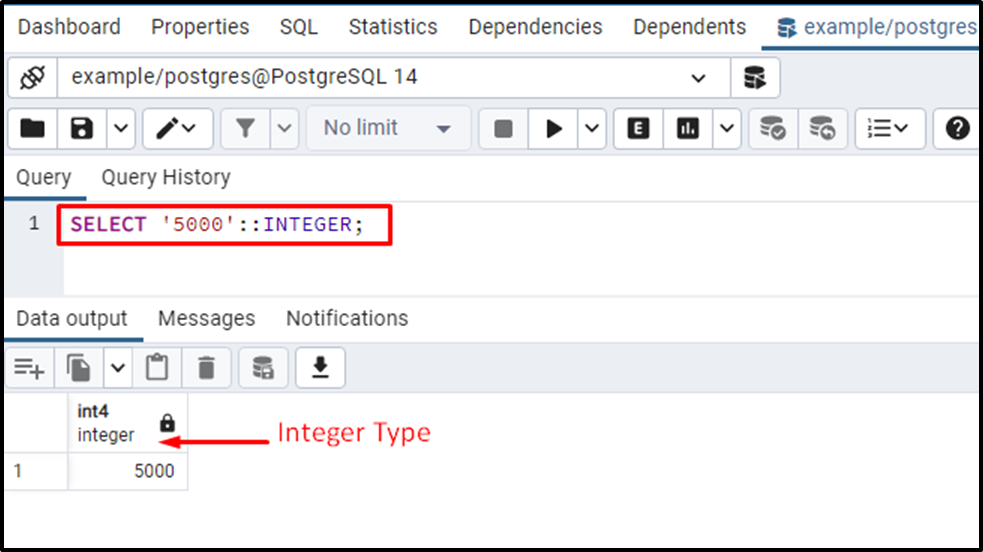The output proved that the given string had been successfully converted into the integer type.

Example #2: How to Use the :: Operator on Table’s Data?

In this example, we will calculate the sum of the emp_salary column. But to do that, firstly, we will utilize the “::” operator to convert the emp_salary column into integer data type, and then we will perform addition on that column:

```SELECT SUM(emp_salary :: INTEGER)
AS total
FROM emp_data;```

The above query performs the following functionalities:

- The :: operator will convert the column’s type from string to int.

- The SUM() function will perform addition on the emp_salary column.

Following will be the resultant outcome: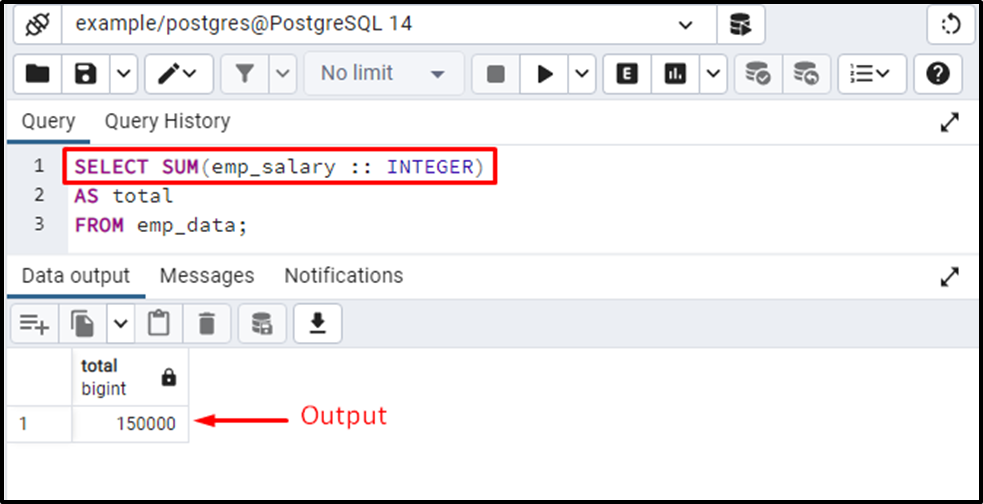Output proved that this time the SUM() function succeeded in finding the sum of the selected column.

How to Use CAST Operator for String to Double Conversion?

In Postgres, if we specify the below-given syntax:

`SELECT CAST ('expression' AS DOUBLE);`

We will encounter an error “double doesn’t exist”.

Example #1: How Does the CAST Operator Work in PostgreSQL?

Let’s first use the “DOUBLE” as a data type to convert the ‘5000.005’ to the double type:

`SELECT CAST ('5000.005' AS DOUBLE);`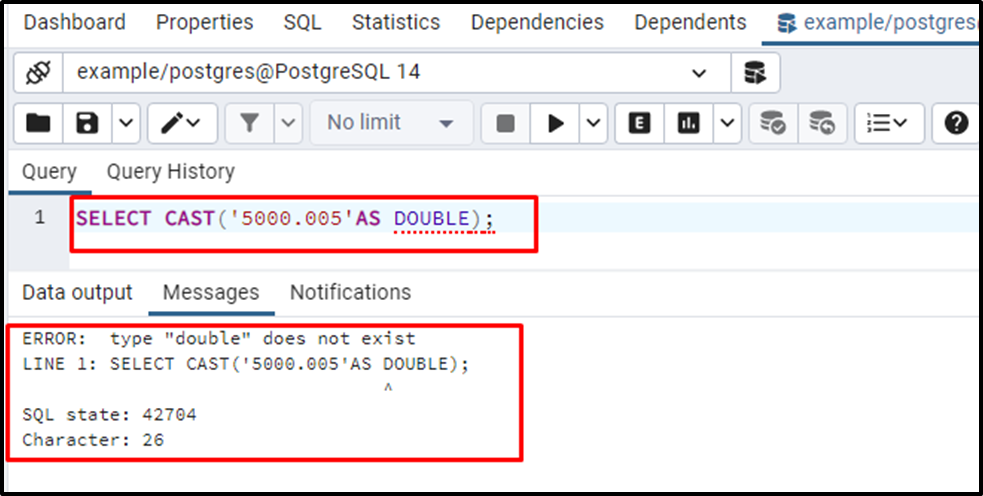The output verified that PostgreSQL generated an error when we tried to convert the given string into the double type.

Therefore, to convert a string into a double type, we must use the “DOUBLE PRECISION” as a data type as shown below:

`SELECT CAST ('expression' AS DOUBLE PRECISION);`

Example #2: How to Use the CAST Operator to Perform Conversion From String to Double Precision?

Let’s specify the “DOUBLE PRECISION” as a conversion type to convert the given string into double precision:

`SELECT CAST('5000.005' AS DOUBLE PRECISION);`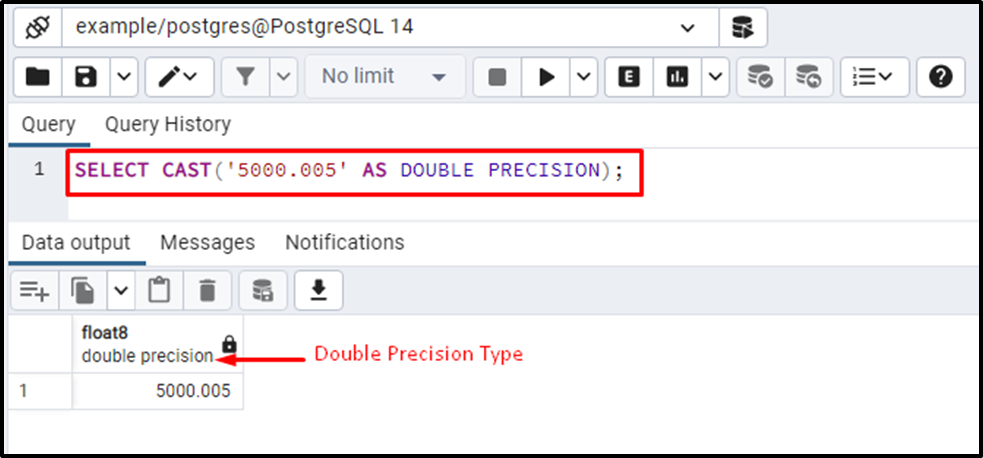The output clarifies that the string “5000.005” has been converted into the double precision type successfully.

How to Perform String to Double Conversion Using ‘::’ Operator in PostgreSQL?

The “::” will throw a “double doesn’t exist” error if we specify “DOUBLE” as a conversion type. So, we will use the DOUBLE PRECISION as a conversion type to convert the given string into a double type value:

`SELECT 'expression' :: DOUBLE PRECISION;`

Example: How to Use the ‘::’ Operator to Convert a String Into a Double Precision Value?

Let’s execute the below statement to convert “5000.005” to a double precision value:

`SELECT '5000.05' :: DOUBLE PRECISION;`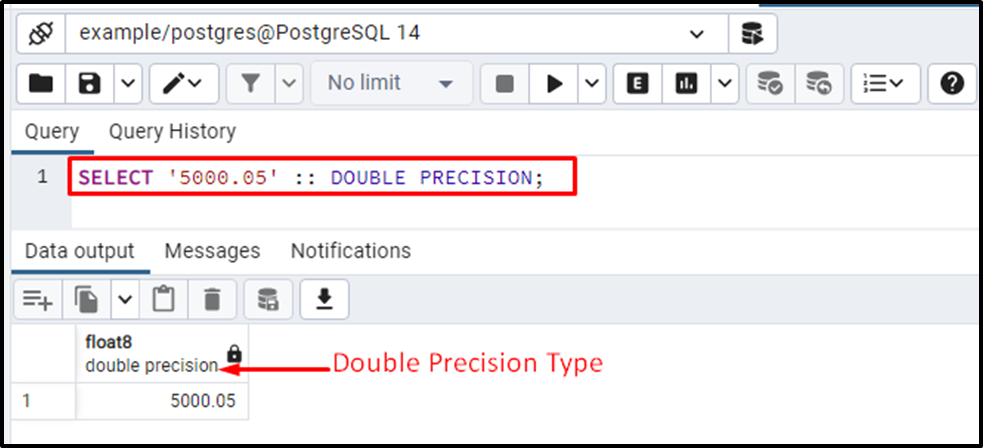The output authenticates that the string “5000.005” has been successfully converted into the double precision type.

How to Use TO_NUMBER Function in Postgres?

In Postgres, TO_NUMBER() is a built-in function that converts a string/text into a number. The syntax of the TO_NUMBER() is given below:

`TO_NUMBER(str, format);`

Here, “str” represents a string that will be converted into a number based on the specified “format”. There are multiple valid formats for the TO_NUMBER() function that can be used according to the given situation. You can learn more about the valid formats of the TO_NUMBER() function at the following link.

Example: How Does the TO_NUMBER() Function Work in PostgreSQL?

Let’s convert the “-1214.72” into a number using TO_NUMBER():

`SELECT TO_NUMBER('-1214.72', 'S9999D99');`

In this example, “-1214.72” is a string to be converted while “S9999D99” is a format based on which the given string will be converted. Here is the detailed description of the specified format:

- S represents a sign (i.e. plus or minus).

- Four 9’s represent that there are four digits after the specified sign.

- The D symbol after the four 9’s represents that there are four digits after the specified sign (i.e. minus).

- The last two 9’s represent that there are two more digits after the fraction/period.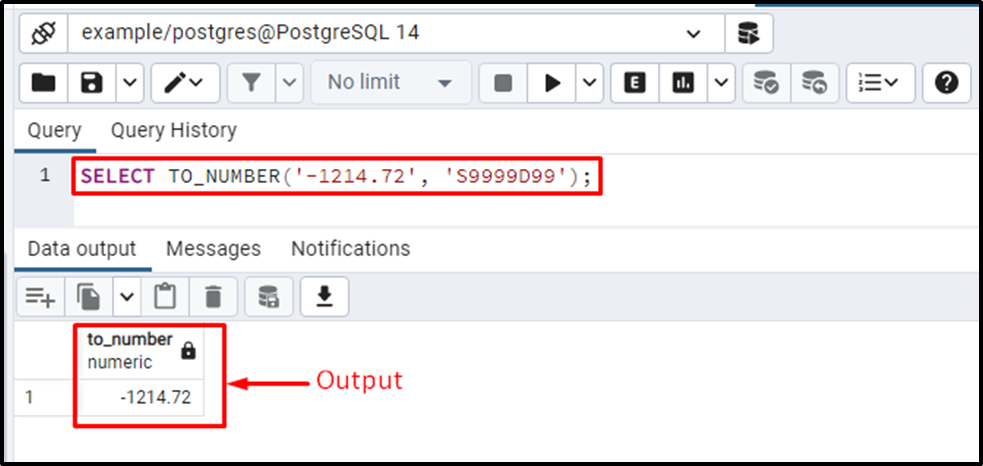The output shows that the TO_NUMBER() function successfully converted the given string into a number.

Conclusion

Using the TO_NUMBER(), CAST(), and “::” operator, we can convert a string into a numeric type such as an integer, double, or decimal. Specify a string followed by the “::” operator and then write the targeted type to convert the given string into the targeted data type, such as integer, decimal, or double precision. Or Use the CAST operator with the collaboration of the AS clause to convert a string to a number like an integer, decimal, or double. This post considered different examples for the string-to-number conversion in PostgreSQL.